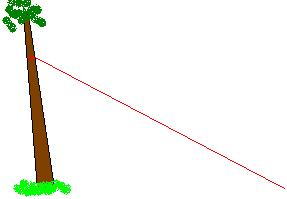Line Pull CalculatorHi Don, Assumptions: Tree is hinged at ground level, it has been cut off! Let T = tension in rope in pounds W = weight of tree in pounds H = distance from hinge (ground) to where rope is tied to tree as measured along the tree Theta = angle between tree and ground alpha = angle between rope and tree L = distance from ground to the center of gravity as measured along the tree, this is the point where a cable attached to the tree could lift it in a balanced position???????? Sum torques about the hinge (butt of tree) T = L*W*cos(theta)/( H*sin(alpha)) Checks: IF theta = 90 degrees the tree will fall over Thus T=0 IF alpha= 90 degrees the tension is a minimum The larger H the smaller the tension Hope this helps, Mitch

 Distance From Ground to Center of Gravity (feet) Tree Weight(pounds) Angle of Tree to Ground Height Rope is Tied(feet) Angle of Tree to Rope